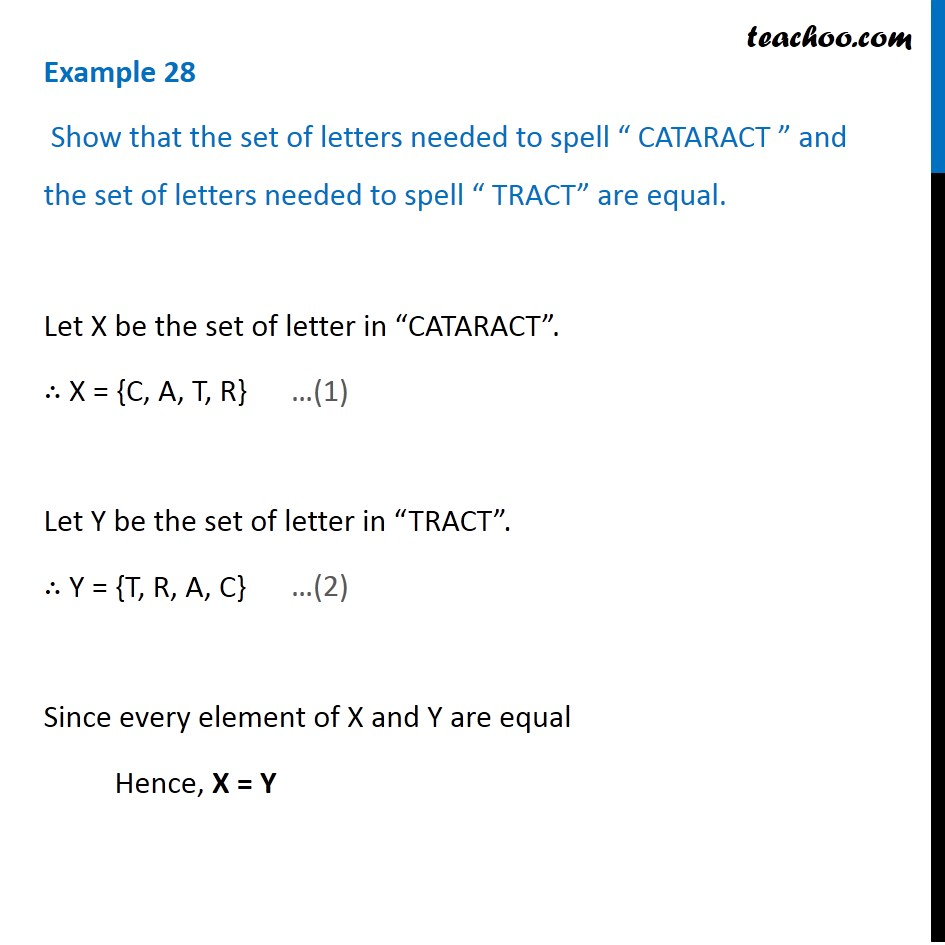1. Chapter 1 Class 11 Sets (Term 1)
2. Serial order wise
3. Examples

Transcript

Example 28 Show that the set of letters needed to spell “ CATARACT ” and the set of letters needed to spell “ TRACT” are equal. Let X be the set of letter in “CATARACT”. ∴ X = {C, A, T, R} Let Y be the set of letter in “TRACT”. ∴ Y = {T, R, A, C} Since every element of X and Y are equal Hence, X = Y

Examples